Understanding Instrument Specifications -- How to Make Sense Out of the Jargon

Überblick

This tutorial is part of the National Instruments Measurement Fundamentals series. Each tutorial in this series will teach you a specific topic of common measurement applications by explaining theoretical concepts and providing practical examples.

For many years, multifunction data acquisition boards have given engineers and scientists an interface between transducers and computers. These boards, which are designed for a wide range of applications, are used in a variety of applications from dynamometer control to CD player testing. Because of developments in component and computer technologies, it is now possible to build computer-based measurement systems that rival -- and at times exceed -- the performance of systems based on traditional boxed instruments. Traditionally, data acquisition boards and stand-alone instruments have been specified by using either different terminology or similar terminology with different meanings. This application note resolves this issue by providing clear definitions for specification parameters such as NMRR, CMRR, and ECMRR (just to name a few) and illustrates how these specifications directly influence your measurements.

We begin by providing a strictly technical definition for each of the key parameters that you are likely to come across when specifying your measurement system. We then cover some common mistakes and conclude with a suggested error budget template.

Definitions

•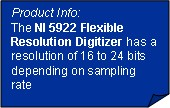Resolution -- the smallest amount of input signal change that the instrument can detect reliably. This term is determined by the instrument noise (either circuit or quantization noise). For example, if you have a noiseless voltmeter that has 5 1/2 digits displayed and is set to the 20 V input range, the resolution of this voltmeter is 100 µV. This can be determined by looking at the change associated with the least significant digit.
Now, if this same voltmeter had 10 counts of peak-to-peak noise, the effective resolution would be decreased because of the presence of the noise. Because of the Gaussian distribution of the noise, in this case the effective resolution would be 0.52 ´ 1 mV. In general when you have a measurement system that has X counts of Gaussian noise, the effective resolution of the system is given by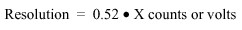• A flicker-free measurement system or device has an effective resolution equal to 1 count.

• Effective Number of Digits (ENOD) -- a performance parameter for an instrument or digitizer, defined in terms of the total range and the resolution: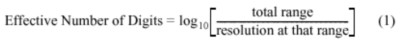ENOD = log10((20-(-20))/0.52 mV) = 4.886 for the noisy meter given in the example above, and for the noiseless instrument ENOD = log10((20-(-20))/100 µV) = 5.60206.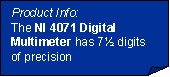Note: Peak-to-peak = rms x 6.6 for agreement with mathematical formulas.

• Digits Displayed and Overranging -- the number of digits displayed by the readout of a DMM. It is often specified as a certain number of full digits (i.e. digits that can display values from 0 to 9) and an additional overrange digit referred to as a 1/2 digit. That 1/2 digit typically shows only the values 0 or 1. For example, a 6 1/2 digit display has a 7-digit readout, but the most significant digit can read 0 or 1 while the other 6 digits can take any value from 0 to 9. Hence, the range of counts is ±1,999,999. This should not be confused with resolution; a DMM can have many more digits displayed than its effective resolution.

Note: The 1/2 digit has been referred to by DMM manufacturers as any digit that is not a full digit. A full digit can take any value from 0 to 9.

• Number of Counts -- the number of divisions into which a given measurement range is divided. For example, a traditional 5 1/2 digit voltmeter has ±199,999 counts (from +199,999 to -199,999) or 399,999 total counts. The weight of a count is given by the following expression (2):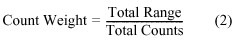• Number of Least Significant Bits -- the number of divisions into which a given measurement range is divided. For example, a 12-bit digitizer has 4096 LSBs. (In a bipolar 12-bit system, the range of returned codes is typically -2048 to 2047.) The weight of an LSB is given by the following formula (3):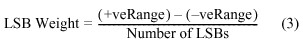and the number of LSBs for an n-bit digitizer is given by (4)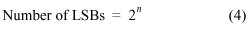Note: LSBs and counts are the same. A non-DMM digitizer usually refers to its counts as the number of LSBs. This definition is given here for comparison purposes.

• Sensitivity -- a measure of the smallest signal the instrument can measure. Usually, this is defined at the lowest range setting of the instrument. For example, an AC meter with a lowest measurement range of 10 V may be able to measure signals with 1 mV resolution but the smallest detectable voltage it can measure may be 15 mV. In this case, the AC meter has a resolution of 1 mV but a sensitivity of 15 mV.

• Accuracy -- a measure of the capability of the instrument to faithfully indicate the value of the measured signal. This term is not related to resolution; however, it can never be better than the resolution of the instrument. The accuracy is often specified as: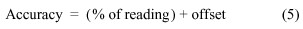For example, a 5 1/2 digit voltmeter can have an accuracy of 0.0125% of reading + 24 µV on its 2.5 V range which results in an error of 149 µV when measuring a 1V signal. On the other hand, the resolution of this same voltmeter is 12 µV, 12 times better than the accuracy. Keep in mind that the accuracy of your measurement is affected by several factors and we will discuss these factors later in this paper.

• Precision -- a measure of the stability of the instrument and its capability of resulting in the same measurement over and over again for the same input signal. It is given by: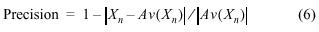where Xn = the value of the nth measurement
and Av(Xn) = the average value of the set of n measurement.

For instance, if you are monitoring a constant voltage of 1 V, and you notice that your measured value changes by 20 µV between measurements, for example, then your measurement precision is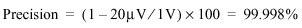which is 6.25 times better than the voltmeter accuracy. This specification is most valuable when you are using the voltmeter to calibrate a device or performing relative measurements.

• Normal Mode -- an indication of a differential change at the inputs of the measuring instrument (see Figure 1).

• Common Mode -- an indication of an equal change on both inputs of the measuring instrument (see Figure 1).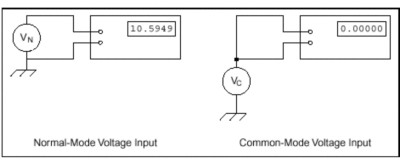Figure 1. Normal-Mode and Common-Mode Applied Voltages

• Normal-Mode Rejection Ratio (NMRR) -- describes the ability of the instrument to reject a normal (differential) signal, it is given by the following formula: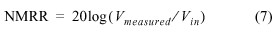where Vin is applied differentially to the instrument inputs, and Vmeasured is the value indicated by the DMM. This specification is useful for measurement systems that have filters to eliminate signals at a given frequency or over a range of frequencies. For systems that do not have filters, the NMRR is 0 dB. This specification, which is often used to indicate the capability of the instrument to reject 50 or 60 Hz, is valid only at the specified frequency and useful only when making DC measurements.

For example, if you are measuring 1 mVDC with a DMM that specifies a NMRR of 130 dB at 60 Hz, and you have a normal-mode interference (noise) of 100 mVrms, then your resulting measurement error is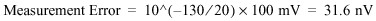which is 0.003 percent of your measured signal instead of the 10,000 percent error that the 100 mV interference implies.

• Common-Mode Rejection Ratio (CMRR) -- a measure of the capability of an instrument to reject a signal that is common to both input leads. For instance, if you are measuring a thermocouple in a noisy environment, the noise from the environment appears on both input leads. Therefore, this noise is a common-mode signal that is rejected by the CMRR of the instrument. The CMRR is defined by the following equation: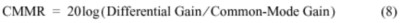This specification is very important because it indicates how much of the common-mode signal will affect your measurement. CMRR is also frequency dependent. An equivalent equation to represent CMRR is as follows: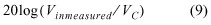where Vinmeasured is the indicated value for an applied common-mode voltage VC

• Effective Common-Mode Rejection Ratio (ECMRR) -- only valid for DC measurements, it is the sum of CMRR and NMRR at a given frequency. It is the effective rejection on a given noise signal that is applied to both input leads because it is rejected first by the CMRR capability of the instrument and then again by its NMRR capability. This specification is mostly useful at the power line frequencies, where most of the noise resides.

• Nonlinearity -- the amount of distortion that is applied on the signal. This distortion, varies with signal input level and or signal frequency. If we went back and looked at the accuracy specifications, we would notice that this specification is based on the assumption that the instrument has a transfer function described as follows: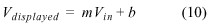In this accuracy specification, the percent and offset terms apply to the degree of accuracy to which we know the slope m and intercept b of the transfer function. However, many measurement systems have transfer functions that are more accurately modeled as 2nd and 3rd order polynomials. To keep the calibration simple the transfer function is assumed to be linear and a nonlinearity error is specified to compensate for the 2nd or 3rd order part of the transfer function. This error is given as a function of percent of range and not of reading. The reason for this is that the peak nonlinearity error could occur at any point over the full-scale input range. For example, given a nonlinearity of 0.0015% and a range of 2 V, the voltmeter has an additional error of 0.0015%×2 = 30 µV. This error is sometimes lumped in the offset error indicated in the accuracy table; be careful not to count it twice in your error budget.

• Crest Factor -- the ratio of the peak voltage to rms voltage of a signal, which is given by the following formula: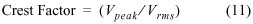For a sine wave the crest factor is 1.414, for a square wave the crest factor is 1. This specification is important because it indicates the maximum peak value of an arbitrary waveform that the DMM can handle without overloading. The crest factor also affects the accuracy of the AC measurement. For example, given a certain DMM with an AC accuracy of 0.03% (this is always specified for sine waves), and has an additional error of 0.2% for crest factors between 1.414 and 5, then the total accuracy for measuring a triangular wave (crest factor = 1.73) is 0.03% + 0.2% = 0.23%.

• Root Mean Square (rms) -- a value assigned to an AC signal that represents the amount of DC signal required to produce an equivalent amount of heat in the same load. Its mathematical equation is given by: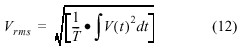or simplified to: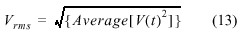• True rms -- a specific method of measuring the rms value of a signal. This method results in the most accurate rms value regardless of the shape of the waveform. Other methods of measuring rms values exist, such as the rectifier or mean absolute deviation method; however, these methods are accurate only for sine wave signals.

• 2-Wire Resistance Measurement -- a method of measuring resistance that uses only two test leads. To measure resistance, the voltmeter typically passes a current through the resistor of interest and then measures the voltage developed across this resistor. In this method, both the injected current and the sensed voltage use the same pair of test leads, as indicated in Figure 2.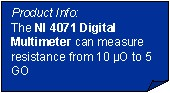• 4-Wire Resistance Measurement -- a method of measuring resistance that uses four test leads. One pair is used for the injected current, the other pair is used for sensing the voltage across the resistor. This more accurate method is recommended for measuring resistance below 100 ohms. The improved accuracy is achieved because the test leads resistances are removed from the measurement path as indicated in Figure 2.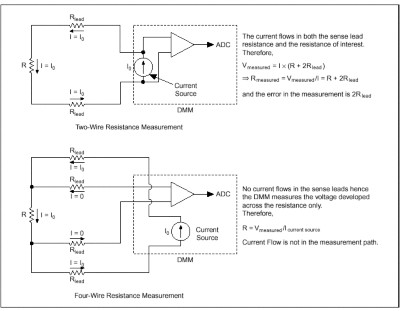Figure 2. Two-Wire and Four-Wire Resistance Measurement Methods

Resolution, Precision, Accuracy

Often the terms resolution, precision, and accuracy are used interchangeably, but they actually indicate very different entities, as we discussed in the previous section. Although common sense indicates that a 6 1/2 digit multimeter must be accurate to the 6 1/2 digit level, this may not be the case. The number of digits simply relates to the number of figures that the meter can display and not to the minimum distinguishable change in the input (refer to Digits Displayed and Overranging definition above). Therefore, when using or evaluating an instrument, remember that the number of digits may refer to the display and not directly to the resolution of the instrument.

You need to verify that the instrument sensitivity and effective resolution are enough to guarantee that the instrument will give you the measurement resolution you need. For example, a 6 1/2 digit multimeter can represent a given range with 1,999,999 counts or units. But if the instrument has a noise value of 20 counts peak to peak, then the minimum distinguishable change must be at least 0.52 ´ 20 counts. Referring to equation (4) above, the effective number of digits is: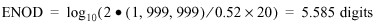Often, this error is added in the DMM specifications under Percent Range, where the source of the error -- nonlinearity, noise, or offset -- is not identified.

This aspect of the technical specifications relates to the first digital multimeters, which had a limited number of digits displayed to keep the cost of the instrument as low as possible. With the advent of more sophisticated digital instruments and, ultimately, of virtual instruments, the cost of the instrument display is no longer an issue. Therefore, care must be taken in specifying the number of digits of a measurement device (whether computer-based, PXI/CompactPCI, VXI, or GPIB controlled). The resolution, accuracy, nonlinearity, and noise of the measurement device must be considered when determining the number of digits to display to the user. For example, consider an instrument that uses a 24-bit analog-to-digital converter (ADC) and can display seven digits of data (seven 9s). However, if the six least-significant bits are noisy and thus not carrying any valuable information, the resolution of this ADC is reduced to 18 bits (five digits) and the instrument vendor should display no more than five digits.

The NI 4050 and NI 4060 digital multimeters and the NI 4350/4351 temperature and voltage instruments are based on a 24-bit ADC and provide 24-bit data (7 digits). However, following these guidelines, the information returned to the user has been voluntarily limited to 5 1/2 digits (18.6 bits) to maintain the correct relationship between the number of digits displayed and the effective resolution of the instrument. Because National Instruments has adopted the definition of resolution listed at the beginning of this document, the number of digits displayed matches the instrument resolution.

Sometimes it is difficult to make a clear distinction between precision of the instrument and its accuracy. Precision,which relates to the repeatability of the measurement, is determined by noise and short-term drift of the instrument. (Long-term drift of an instrument affects precision only if it is considered over extended period of time.) The precision of an instrument is often not provided directly, but it must be inferred from other specifications such as the 24 hour ±1 °C specification, the transfer ratio specification, noise, and temperature drift. Precision is meaningful primarily when relative measurements (relative to a previous reading of the same value) need to be taken -- a typical example is device calibration.

Accuracy of an instrument is absolute and must include all the errors resulting from the calibration process. It is interesting to note that sometimes the accuracy specifications are relative to the calibration standard used. In such a case, it is important to include in your error budget the additional errors due to this calibration standard.

In the following section we will go through an error budget calculation to determine the instrument total accuracy.

Error Calculation or Accuracy Determination

DC Measurement

 Error Type Reading-Dependent Errors Noise and Range-Dependent Errors Specified Accuracy % of reading x reading/100 offset Nonlinearity% nonlinearity x range/100 System Noiserms noise x 6.6 (to find peak-to-peak value) Settling Time % settled x Step change/100 (this must be added for scanning systems unless it is included in the accuracy specifications)NM NoiseNormal Mode Noise x 10^(-NMRR/20) CMVCommon mode Voltage x 10^(-CMRR/20) Temperature Drift (this must be added if your temperature range is outside the specified range for the given accuracy tables) (% of reading/°C) x X °C x reading/100 X is the temperature difference between the specified temperature range and the actual operating temperature. (offset /°C) x X °C X is the temperature difference between the specified temperature range and the actual operating temperature.

Let us consider the National Instruments NI 4350 Series 5 1/2 digit Temperature and Voltage Data Logger. We will calculate the total accuracy of a 1 V reading. Let us also assume that the instrument is at an ambient temperature between 15 and 35 °C, and it has been less than a year since the last calibration was performed but more than 90 days. The total accuracy based on the error budget determined above is:

 Error Type Percent of Reading Errors Range-Dependent Errors Specified Accuracy 1 V x 0.0131% = 131 mV 3 µV Nonlinearity0, included in accuracy System Noise0, included in offset Settling Time not needed as specification table included any errors due to scanningNM Noise, assume 1 mVrms of environmental noise1 mV x 1.4 x l0^(-100/20) = 0.01 µV CMV, assume maximum CMV of 2.5 V2.5 x 10^(-100/20) = 25 µV Temperature Drift N/A (because the NI 4350 specification tables cover 15 to 35 °C) N/A (because the NI 4350 specification tables cover 15 to 35 °C) Subtotal 131 µV 28.01 µV Total Maximum Error 159.01 µV or 0.016% of reading

AC Measurement

 Error Type Reading-Dependent Errors Range-Dependent Errors Specified Accuracy at a Given Signal Frequency Range % of reading x reading/100 offset Nonlinearity% nonlinearity x range/100 System Noiserms noise x 3.46 (to find peak to peak value x assume gaussian noise) Settling Time % settled x Step change/100 (this must be added for scanning systems unless it is included in the accuracy specifications)CMVCommon mode voltage x 10^(-CMRR/20) Temperature Drift (this must be added if your temperature range is outside the specified range for the given accuracy tables) (% of reading/°C) x X °C x reading/100 X is the amount of temperature drift from the specified temperature range. offset /°C x X °C X is the amount of temperature drift from the specified temperature range. Crest Factor Error X x reading/100 Add X% additional error based on the type of waveform.Let us consider the NI 4050 5 1/2 digit multimeter and calculate the total accuracy of a 1 Vrms reading. Let us also assume that the instrument is at an ambient temperature between 15 and 35 °C, and it has been one year since the last calibration was performed. Because this is an AC measurement, we need to specify the frequency of the signal measured and the crest factor. Let us assume a frequency of 1 kHz and a crest factor of 2. The total accuracy based on the error budget determined above is:

 Error Type Reading-Dependent Errors Range-Dependent Errors Specified Accuracy at a Given Signal Frequency Range 0.42% x 1 V = 4.2 mV 1.2 mV Nonlinearity Included in specification table Included in specification table System NoiseIncluded in specification table Settling Time This is not a scanning DMM and let us assume that the signal is not changingCMV assume maximum allowable CMV of 250 V250 ´ 10^(-100/20) = 2.5 mV Temperature Drift Not applicable because the temperature range is included in the specification tableCrest Factor Error 0% x 1 V/100 = 0 mVSubtotal 4.2 mV 3.7 mV Total Maximum Error 7.9 mV or 0.79% of reading

Conclusion

When considering a DMM or a virtual instrument, it is important to have a clear understanding of all the parameters involved in defining the characteristics of the measurement device. The number of digits listed in the data sheet is an important piece of information, but it should not be considered the ultimate or only parameter to take into account. By knowing the accuracy and resolution requirements for your application, you can compute the total error budget of the measurement device you are considering and verify that it satisfies your needs. Do not hesitate to ask a vendor to clarify the meaning of the specifications in a data sheet. Not knowing the true performance of your instrument could lead you to incorrect readings, and the cost of this error could be very high.

Relevant NI Products

Customers interested in this topic were also interested in the following NI products:

For more tutorials, return to the NI Measurement Fundamentals Main page.